# Introduction

Subsets play an important role in almost any data analysis. Imagine a data set of countries, with variables named `population`, `continent`, `landlocked`, and a variety of other variables representing economic and political characteristics. We might wish to examine subsets of the data set based on the `continent` variable. Within each of these subsets, we might wish to examine nested subsets based on the `population` variable, for example, countries with populations under 30 million and over 30 million. We might continue to a third level of nesting based on the `landlocked` variable. Nested subsets help us to answer questions like the following: Among African countries with a population over 30 million, what percentage are landlocked?

Even in simple situations like this, it can be a chore to keep track of nested subsets and to calculate percentages. And as the number of subsetting variables increases, the number of nested subsets grows rapidly. When there are missing values in the data set, the task becomes even more complicated. For these reasons, it is best not to perform nested subsetting by hand.

Nested subsets arise in many situations. Consider, for example, flow diagrams for clinical studies. In a typical study, participants are a subset of eligible patients who in turn are a subset of patients who were assessed. Because mistakes inevitably arise during manual calculation and transcription, it is not uncommon for flow diagrams in published studies to contain errors. And although the errors that make it to publication are often small, they can occasionally be disastrous.

A solution to the problem of calculating nested subsets and displaying information about them is presented here. The idea is to represent nested subsets of a data set (where the subsets are defined by specific variables in the data set), using a tree structure, which we call a variable tree. `vtree` is a flexible tool for calculating and drawing variable trees. It can be used to explore a data set, to construct figures for reports and publications, and to double-check code to ensure that subsetting has been performed correctly.

## Basic features of a variable tree

The examples that follow use a data set of 46 fictitious patients called `FakeData`. Based on this data set, the variable tree below depicts subsets defined by `Sex` (M or F) nested within subsets defined by disease `Severity` (Mild, Moderate, Severe, or NA). Later we’ll see how variable trees with more than two variables are particularly useful.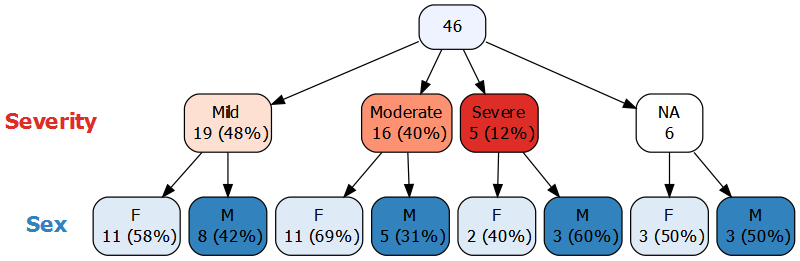A variable tree consists of nodes connected by arrows. At the top of the diagram above, the root node of the tree contains all 46 patients. The rest of the nodes are arranged in successive levels, where each level corresponds to a variable. Note that this highlights one difference between variable trees and some other kinds of trees: at each level of a variable tree, regardless of the branch, the nodes represent values of the same variable. In contrast, consider decision trees, which can have splits on different variables at the same level.

Continuing with the variable tree above, the nodes immediately below the root represent values of `Severity` and are referred to as the children of the root node. In this case, `Severity` was missing (NA) for 6 patients, and there is a node for these patients. Inside each of the nodes, the number of patients is displayed and—except for in the missing value node—the corresponding percentage is also shown. Note that, by default, `vtree` displays “valid” percentages, i.e. the denominator used to calculate the percentage is the total number of non-missing values, 40.

The nodes in the next level (which is the final level for this tree) correspond to values of `Sex`. These nodes represent males and females within subsets defined by each value of `Severity`. In each of these nodes the percentage is calculated in terms of the number of patients in its parent node.

Like any node, a missing-value node can have children. For example, of the 6 patients for whom `Severity` is missing, 3 are female and 3 are male. By default, `vtree` displays the full missing-value structure of the specified variables in the data frame.

Also by default, `vtree` automatically assigns a color palette to each variable. `Severity` has been assigned red hues (lightest for Mild, darkest for Severe), while `Sex` has been assigned blue hues (light blue for females, dark blue for males). The node representing missing values of `Severity` is colored white to draw attention to it.

## Variable trees vs. contingency tables

A tree with two variables is similar to a two-way contingency table. In the example above, `Sex` is shown within levels of `Severity`. This corresponds to the following contingency table, where the percentages within each column add to 100%. These are called column percentages.

Mild Moderate Severe NA
F 11 (58%) 11 (69%) 2 (40%) 3 (50%)
M 8 (42%) 5 (31%) 3 (60%) 3 (50%)

Likewise, a tree with `Severity` shown within levels of `Sex` corresponds to a contingency table with row percentages.

The contingency table above is more compact than the corresponding variable tree, but some people find the variable tree easier to interpret. When three of more variables are of interest, multi-way contingency tables can be used. These are typically displayed using several two-way tables. In this situation, variable trees are generally easier to interpret.

It is also noteworthy that contingency tables are not always more compact than variable trees. When most cells of a large contingency table are empty (in which case the table is said to be sparse), the corresponding variable tree may be more compact since empty-nodes are not shown.

Variable trees are thus an appealing alternative to multi-way contingency tables and can also be used to display a wide variety of information including:

• multi-way intersections (often shown in Venn diagrams),

• flow diagrams involving a sequence of inclusion/exclusion steps,

• longitudinal events.

## Features of vtree

`vtree` is designed to be quick and easy to use, so that it is convenient for data exploration, but also flexible enough that it can be used to prepare publication-ready figures. To generate a basic variable tree, it is only necessary to provide `vtree` with a data frame and some variable names. However extra features make `vtree` much more useful. `vtree` provides:

## Technical overview

`vtree` is built on open-source software: in particular Richard Iannone’s DiagrammeR package, which provides an interface to the Graphviz software using the htmlwidgets framework. A formal description of variable trees follows.

The root node of the variable tree represents the entire data frame. The root node has a child for each observed value of the first variable that was specified. Each of these child nodes represents a subset of the data frame with a specific value of the variable, and is labeled with the number of observations in the subset and the corresponding percentage of the number of observations in the entire data frame. The nth level below the root of the variable tree corresponds to the nth variable specified. Apart from the root node, each node in the variable tree represents the subset of its parent defined by a specific observed value of the variable at that level of the tree, and is labeled with the number of observations in that subset and the corresponding percentage of the number of observations in its parent node.

Note that a node always represents at least one observation. And unlike a contingency table, which can have empty cells, a variable tree has no empty nodes.

# The `vtree` function

Consider a data frame named `df`, which includes discrete variables `v1` and `v2`. In this case, a variable tree can be displayed using the following command:

``vtree(df,"v1 v2")``

For additional details about how variables can be specified, see the section on specification of variables below.

Numerous additional parameters can be supplied. For example, by default `vtree` produces a horizontal tree (that is, a tree that grows from left to right), but sometimes a vertical tree is preferable. When `horiz=FALSE` is specified, `vtree` generates a vertical tree.

## Mini tutorial

To display a variable tree for a single variable, use the following command:

``vtree(FakeData,"Severity")``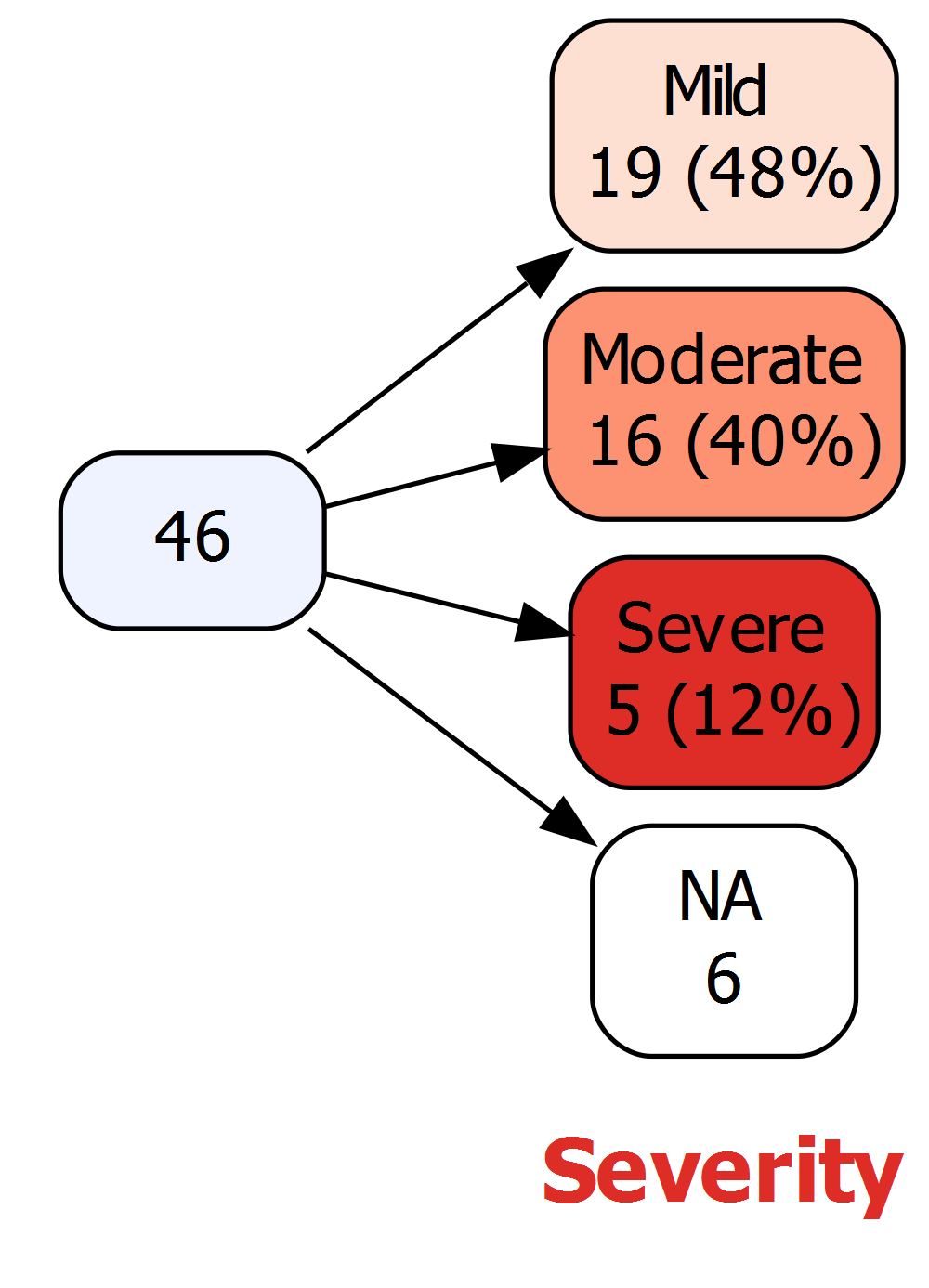Next, consider a vertical variable tree with two variables, `Severity` and `Sex`. A less colorful display with more spacing can be requested by specifying `plain=TRUE`:

``vtree(FakeData,"Severity Sex",horiz=FALSE,plain=TRUE)``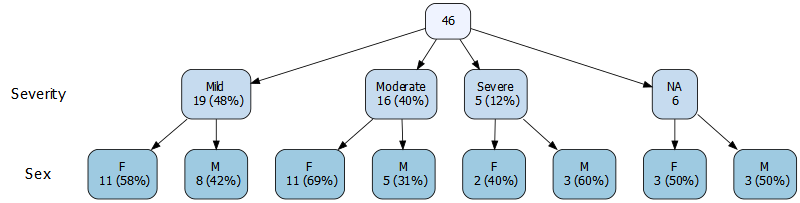At the top, the root node represents the entire data frame. Moving down, each subsequent level of the tree corresponds to a different variable (first `Severity`, then `Sex`). Within each level, each node represents the subset of its parent node where the variable has a specific value. For example, the level for `Severity` has nodes Mild, Moderate, Severe, and NA (which represents missing values). Displayed in each node is the number of observations and (except in the NA node) the conditional percentage, i.e. the number of observations in the node expressed as a percentage of the observations in its parent node.

### Percentages

By default, “valid percentages” are shown, i.e. the denominator is the total number of non-missing values. In the case of `Severity`, there are 6 missing values, so the denominator is 46 - 6 , or 40. There are 19 Mild cases, and 19/40 = 0.475 so the percentage shown is 48%. No percentage is shown in the NA node since missing values are not included in the denominator.

Alternatively, if you don’t want to use valid percentages, specify `vp=FALSE`, and the denominator will be the total number of observations, including any missing values. In this case, a percentage is shown in each of the nodes, including any NA nodes.

If you don’t wish to see percentages, specify `showpct=FALSE`, or if you don’t need to see counts, specify `showcount=FALSE`.

### Displaying a legend and hiding node labels

To include a legend, specify `showlegend=TRUE`. Next to each level of the tree, the variable name is displayed together with color discs and the values they correspond to. For each of the values, overall (marginal) counts are shown, together with percentages.

When the legend is shown, the node labels become redundant, since the colors identify the values of the variables (although the labels may aid readability). If you prefer, you can hide the node labels, by specifying `shownodelabels=FALSE`:

``vtree(FakeData,"Severity Sex",showlegend=TRUE,shownodelabels=FALSE)``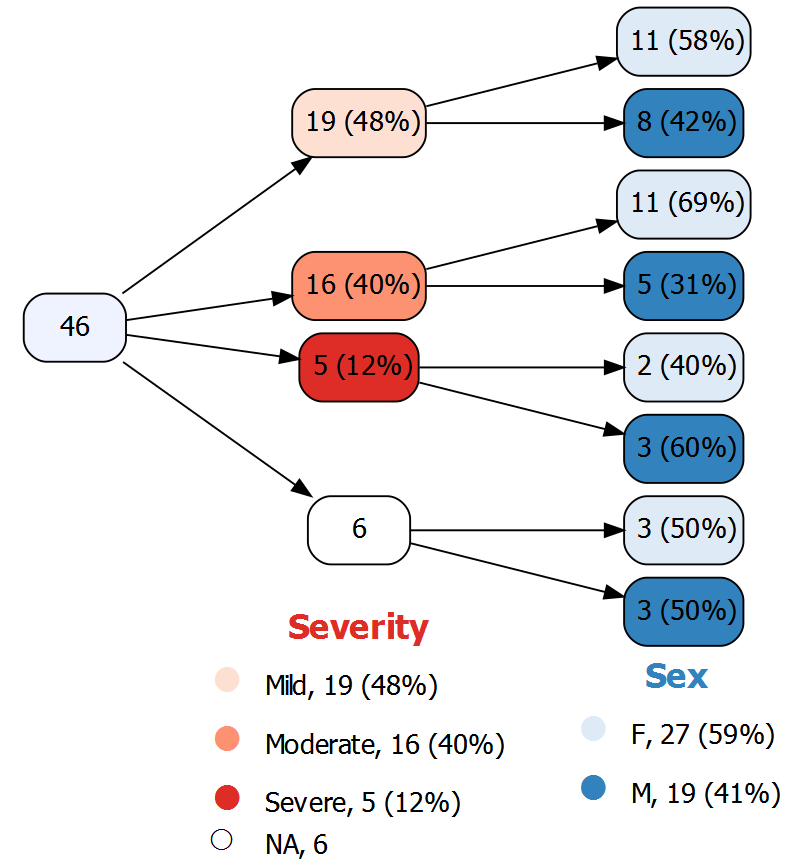The legend shows how colors are assigned to the different values of each variable, and additionally provides marginal (that is, overall) counts and percentages for each variable. Since `Severity` is the first variable in the tree—i.e., it is not nested within another variable— the marginal counts and percentages for `Severity` are identical to those displayed in the nodes. In contrast, for `Sex`, the marginal counts and percentages are different from what is shown in the nodes because the nodes for `Sex` are nested with levels of `Severity`.

(Unfortunately the NA circle in the legend is oddly sized and positioned due to an issue with the corresponding unicode symbols.)

### Putting node labels on the same line as the counts and percentages

When a variable tree is large, it can be difficult to display it in a readable way. One approach that helps is to display the tree horizontally and also to put the node labels on the same line as the counts and percentage by specifying `sameline=TRUE`. For example, the following results in nodes with single-lines labels such as Moderate, 16 (40%), etc.:

``vtree(FakeData,"Severity Sex Viral",sameline=TRUE)``

### Hiding variable names

By default, next to each level of the tree, `vtree` shows the variable name. These can be removed by specifying `showvarnames=FALSE`.

### Text wrapping

By default, `vtree` wraps text onto the next line whenever a space occurs after at least 20 characters. This can be adjusted, for example, to 15 characters, by specifying `splitwidth=15`. To disable line splitting, specify `splitwidth=Inf`. Text wrapping in the legend is controlled independently. To set the splitting in the legend to 8 characters, specify `lsplitwidth=8`. Also note that in the legend, text wrapping can take place not only at spaces, but also at any of the following characters: . - + _ = /

### And more …

This concludes the mini-tutorial. The rest of this vignette details the many features of `vtree`. There is also a section of examples using data from the built-in R datasets package.

## Pruning

Pruning a tree means removing specified nodes along with their descendants. This is useful when a variable tree gets too big, or when you are only interested in certain parts of the tree. For convenience, `vtree` provides several different ways to prune a tree, described below.

### The `prune` parameter

Suppose you don’t want the tree to include individuals whose disease is Mild or Moderate. You can use the `prune` parameter to remove those nodes, and all of their descendants.

The `prune` parameter is specified as a list with an element named for each variable you wish to prune. In the example below the list has one element, named `Severity`. That element in turn is a vector `c("Mild","Moderate")` indicating the values to prune.

``vtree(FakeData,"Severity Sex",prune=list(Severity=c("Mild","Moderate")))``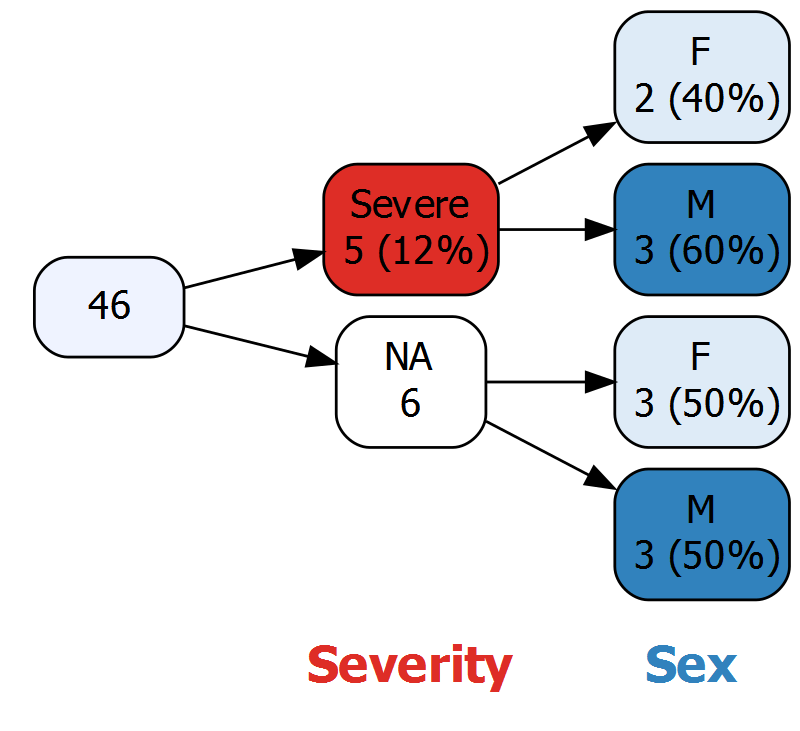Caution: Once a variable tree has been pruned, it is no longer complete. This can sometimes be confusing since not all observations are present at some levels of the tree. It is particularly important to avoid pruning missing value nodes, since this makes it hard to interpret “valid” percentages (i.e. percentages calculated using the number of non-missing observations as denominator).

### The `prunebelow` parameter

A disadvantage of the `prune` parameter is that in the resulting tree, the counts shown in child nodes may not add up to the counts shown in the parent node. For example in the variable tree above, of a total of 46 patients, 5 have Severe disease and `Severity` is unknown for 6. One might wonder what happened to the other 35 patients.

An alternative is to prune below the specified nodes. In this case, this means that the Mild and Moderate nodes will be shown, but not their descendants.

``vtree(FakeData,"Severity Sex",prunebelow=list(Severity=c("Mild","Moderate")))``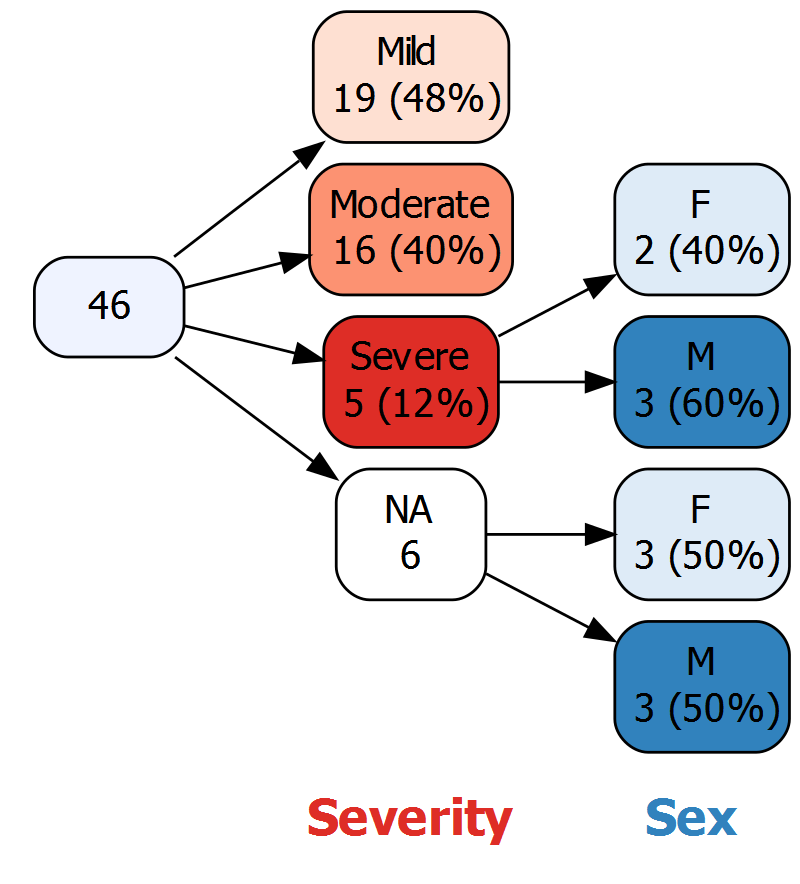### The `keep` and `follow` parameters

Instead of specifying the nodes that should be discarded, sometimes it is more convenient to specify the nodes that should be retained. The `keep` parameter is used to specify nodes that should not be pruned (all other nodes at that level of the tree will be pruned). The `follow` parameter is like the `keep` parameter except that no nodes at that level of the tree will be pruned. Instead, those nodes that are not “followed” will be pruned at the next level.

## Changing how variables and nodes are labeled

By default, `vtree` labels variables and nodes exactly as they are in the data frame. For presentation purposes it is often useful to change these labels.

### Changing variable labels with the `labelvar` parameter

If `Severity` in fact represents severity on day 1, you might want it to appear that way in the variable tree. To do this, use the `labelvar` parameter, which is specified as a vector whose element names are variable names. As an example, if `Severity` in fact represents initial severity, you can specify `labelvar=c(Severity="Initial severity")`.

``vtree(FakeData,"Severity Sex",horiz=FALSE,labelvar=c(Severity="Initial severity"))``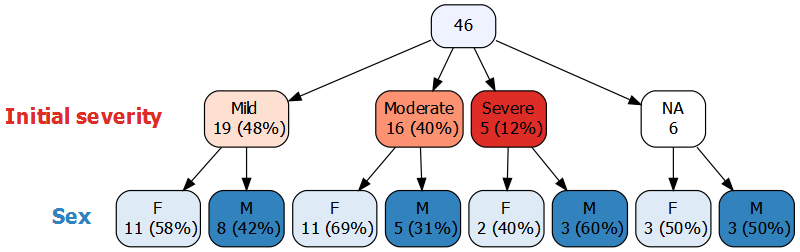### Changing node labels with the `labelnode` parameter

By default, `vtree` labels nodes (except for the root node) using the values of the variable in question. (If the variable is a factor, the levels of the factor are used). Sometimes it is convenient to instead specify custom labels for nodes. You can use the `labelnode` argument to relabel the values. For example, you might want to use “Male” and “Female” instead of “M” and “F”. The `labelnode` argument argument is specified as a list whose element names are variable names. To substitute `New label` for `Old label`, the syntax is: `"New label"="Old label"`. Thus the full specification is: `labelnode=list(Sex=c(Male="M",Female="F"))`.

``vtree(FakeData,"Group Sex",horiz=FALSE,labelnode=list(Sex=c(Male="M",Female="F")))``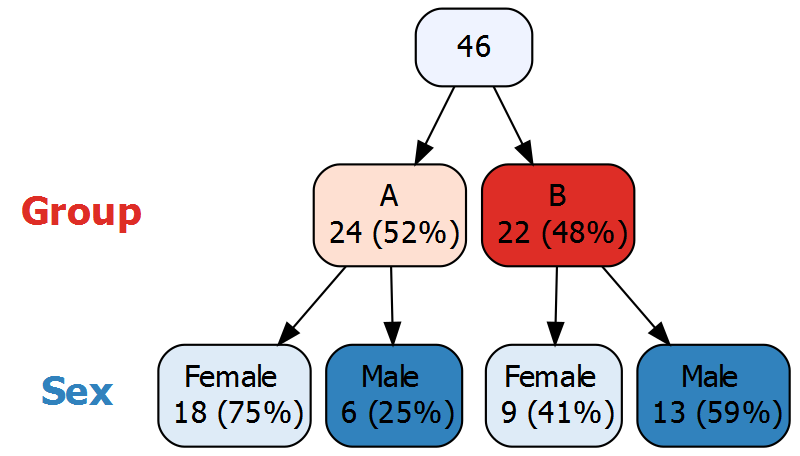### Targeted node labels using the `tlabelnode` parameter

Suppose in the example above that `Group` A represents children and `Group` B represents adults. In `Group` A, we would like to use the labels “girl” and “boy”, while in `Group` B we would like to use “woman” and “man”. The `labelnode` parameter cannot handle this situation because the values of `Sex` need to labeled differently in different branches of the tree. The `tlabelnode` parameter allows “targeted” node labels.

``````vtree(FakeData,"Group Sex",horiz=FALSE,
tlabelnode=list(
c(Group="A",Sex="F",label="girl"),
c(Group="A",Sex="M",label="boy"),
c(Group="B",Sex="F",label="woman"),
c(Group="B",Sex="M",label="man")))``````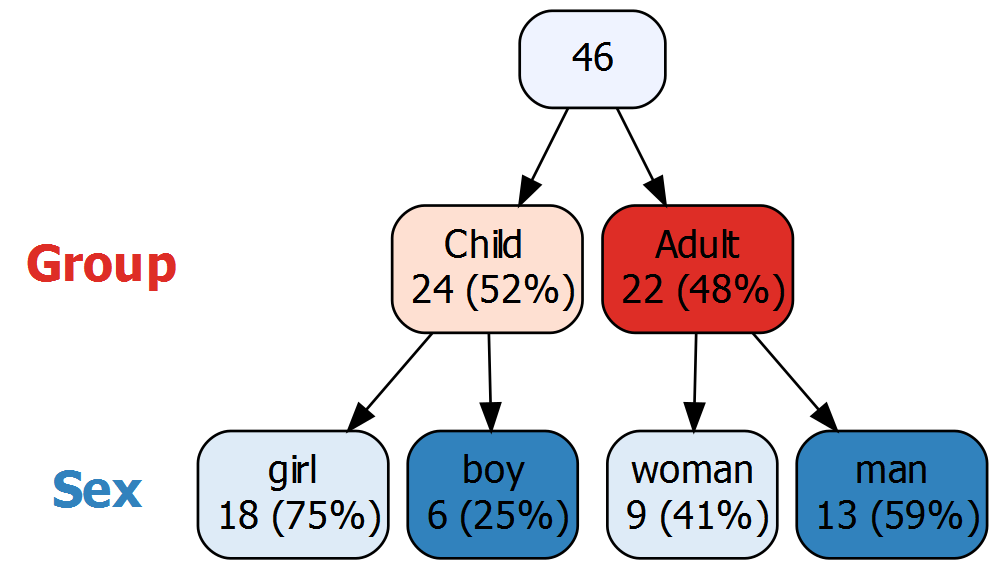## Text and text formatting

`Graphviz`, the open source graph visualization software that provides the basis for `vtree`, supports a variety of text formatting (including boldface, colors, etc.). This is used in `vtree` to control formatting of text such as node labels.

### HTML-like codes for text formatting

NOTE: The section after this one shows how to use an easy alternative to HTML-like codes.

`Graphviz` implements “HTML-like labels”, including:

• `<BR/>` means insert a line break
• `<BR ALIGN='LEFT'/>` means make the preceding line left-justified and insert a line break
• `<I> ... </I>` means display text in italics
• `<B> ... </B>` means display text in bold
• `<SUP> ... </SUP>` means display text in superscript, but note that the font size does not change
• `<SUB> ... </SUB>` means display text in subscript but again note that the font size does not change
• `<FONT POINT-SIZE='10'> ... </FONT>` means set font to 10 point
• `<FONT FACE='Times-Roman'> ... </FONT>` means set font to Times-Roman
• `<FONT COLOR='red'> ... </FONT>` means set font to red

See https://www.graphviz.org/doc/info/shapes.html#html for more details.

Note: To use these HTML-like codes, it is necessary to specify `HTMLtext=TRUE`.

### Markdown-style codes for text formatting

By default, the `vtree` package uses markdown-style codes for text formatting.

• `\n` means insert a line break
• `\n*l` means make the preceding line left-justified and insert a line break
• `*...*` means display text in italics
• `**...**` means display text in bold
• `^...^` means display text in superscript (using 10 point font)
• `~...~` means display text in subscript (using 10 point font)
• `%%red ...%%` means display text in red (or whichever color is specified)

### Adding text to nodes using the `text` parameter

Suppose you wish to add the italicized text “Excluding new diagnoses” to any Mild nodes in the tree. The parameter `text` lets you add text to nodes. It is specified as a list with an element named for each variable. In the example below the list has one element, named `Severity`. That element in turn is a vector `c(Mild="\n*Excluding\nnew diagnoses*")` indicating that the Mild node should include additional text using Markdown-style formatting (i.e. there is a linebreak and the asterisks around the text indicate that it should be displayed in italics):

``````vtree(FakeData,"Group Severity",horiz=FALSE,showvarnames=FALSE,
text=list(Severity=c(Mild="\n*Excluding\nnew diagnoses*")))``````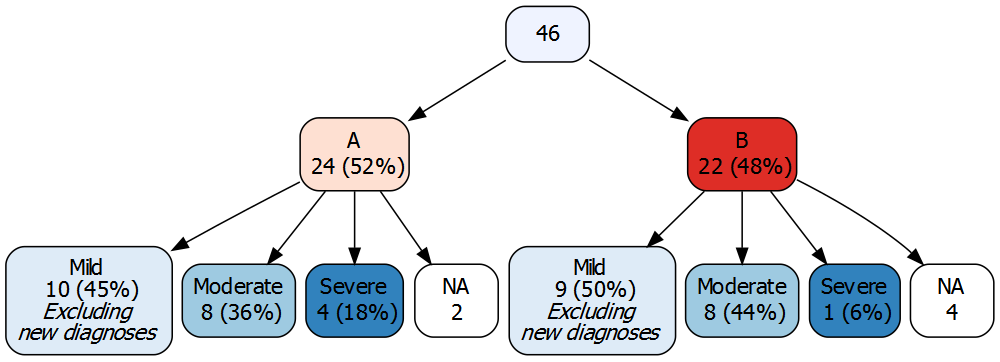### Targeted text using the `ttext` parameter

In the example above, suppose that new diagnoses are only excluded from Mild cases in `Group` B. But the `text` parameter is used to add text to all Mild nodes. Thus, in situations like this, the `text` parameter is not sufficient. Instead, you can use the `ttext` parameter to target exactly which nodes should have the specified text.

The `ttext` parameter requires that you specify the full path from the root of the tree to the node in question, along with the text in question. The `ttext` parameter is specified as a list so that multiple targeted text strings can be specified at once. For example:

``````vtree(FakeData,"Group Severity",horiz=FALSE,showvarnames=FALSE,
ttext=list(
c(Group="B",Severity="Mild",text="\n*Excluding\nnew diagnoses*"),
c(Group="A",text="\nSweden"),
c(Group="B",text="\nNorway")))``````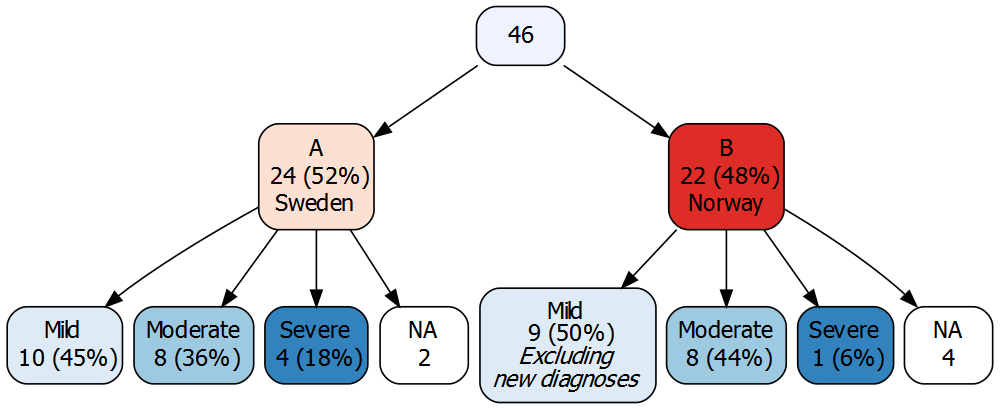## Specification of variables

For convenience, vtree allows you to specify the variable names in a single character string (with the variable names separated by whitespace). If, however, any of the variable names have internal spaces, the variable names must be specified as a vector of character strings.

Additionally, there are several modifiers that can be used to change the way that variables are represented in a tree.

### prefix `is.na:`

If an individual variable name is preceded by `is.na:`, that variable will be replaced by a missing value indicator in the variable tree. (This differs from the `check.is.na` parameter, described below, which is used to replace all of the specified variables with missing value indicators.)

### prefix `stem:`

In datasets exported from REDCap, checkboxes are represented using multiple variables. The `stem:` prefix makes it easier to work with them. This is described in the section on REDCap checkboxes later in this vignette.

### prefix `tri:`

The `tri:` prefix is useful for identifying values of a numeric variable that are extreme compared to the other values in a node. Note: Unlike other variable specifications, which take effect at the level of the entire data frame, the `tri:` prefix takes effect within each node.

The effect of this variable specification is to trichotomize a numeric variable within a node based on the median and the IQR, both computed within that node. The result is three categories:

• “mid”: namely values within plus or minus 1.5×IQR of the median,

• “high”: namely values more than 1.5×IQR above the median,

• “low”: namely values more than 1.5×IQR below the median.

### specification `variable=value`

When a variable takes on a large number of different values, it will result in a very large variable tree. One solution is to prune the tree, for example by keeping just one node. An alternative is to specify the value of the variable that is of primary interest. The result will be to dichotomize the variable at that value. For example if `Severity=Mild` is specified, the `Severity` variable will be dichotomized between `Mild` and `Not Mild`.

### specifications `variable<value`, `variable>value`

These two specifications are used to dichotomize a numeric variable, splitting above and below a specified value. This can be useful for identifying subsets with extreme values.

## Displaying summary statistics in nodes

It is often useful to display information about other variables (apart from those that define the tree) in the nodes of a variable tree. This is particularly useful for numeric variables, which generally cannot be used to build the tree since they have too many distinct values. For example, we might wish to display the mean age for individuals in each node. Or we might wish to list the ID numbers for individuals in each node. The `summary` argument can be used to flexibly specify additional information to display.

### A simple example

The `summary` parameter is specified as a character string that starts with the name of the variable for which a summary is desired. This is followed by a space, and then the rest of the string specifies what to display. Special codes (see table below) are use to indicate the type of summary desired, control which nodes display the summary, etc. For example `%mean%` indicates that the mean of the specified variable should be shown. Thus to display the mean of the numeric variable `Score`, you could specify `summary="Score \nmean score: %mean%"`. Note that the part of the string following the first space is `"\nmean score: %mean%"`. This specifies that in each node, after the usual frequency and percentage, the summary should start on a new line with the words “mean score:” followed by the mean.

``vtree(FakeData,"Severity",summary="Score \nmean score: %mean%",sameline=TRUE,horiz=FALSE)``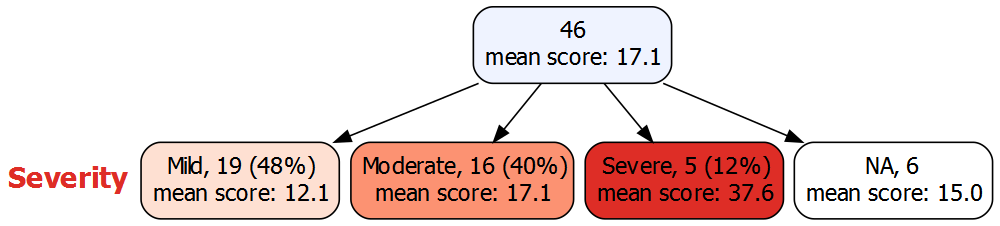The following codes can be used to show summary information:

code result
`%mean%` mean
`%SD%` standard deviation
`%min%` minimum
`%max%` maximum
`%pX%` Xth percentile (e.g. `p50` means the 50th percentile)
`%median%` median, i.e. p50
`%IQR%` IQR, i.e. p25, p75
`%npct%` frequency and percentage of a logical variable. By default “valid percentages” are used. Any missing values are also reported.
`%pct%` same as `%npct%` but percentage only (with no parentheses).
`%list%` list of individual values, separated by commas
`%listlines%` list of individual values, each on a separate line
`%mv%` the number of missing values
`%v%` the name of the variable

The `summary` argument can use any number of these codes, mixed with text and formatting codes.

### More than one variable

Sometimes it is useful to display summary information for more than one variable. To do this, specify `summary` as a vector of character strings:

``````vtree(FakeData,"Severity",horiz=FALSE,showvarnames=FALSE,splitwidth=Inf,sameline=TRUE,
summary=c(
"Score \nScore: mean (SD) %mean% (%SD%)",
"Pre \nPre: range %min%, %max%"))``````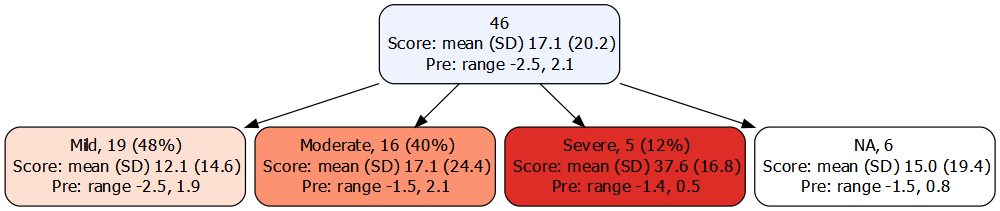### The %npct% code

Suppose we want to know the proportion of patients with a viral infection within each severity level. We could display a variable tree for the variables `Severity` and `Viral`. But that would show a separate node for TRUE and FALSE values of `Viral`, and we only need to know the percentage for the TRUE values. If what we’re looking for is simply the number and percentage of patients with viral infection in each severity group, the `%npct%` code can be used. This results in a simpler tree:

``````vtree(FakeData,"Severity",summary="Viral \nViral %npct%",horiz=FALSE,
showvarnames=FALSE,sameline=TRUE)``````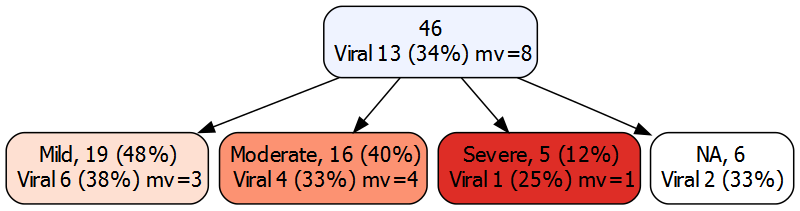Note that in each node, “mv” indicates the number of missing values (if any).

The `%pct%` code is the same as the `%npct%` code except that it does not show the frequency, only the percentage (without parentheses).

### The %list% code

It is sometimes convenient to see individual values of a variable in each node. For example it is often convenient to see ID numbers. To do this, use the `%list%` code. By default this information will be displayed in each node. When a value occurs more than once in the subset, it will be followed by a count of the number of repetitions in parentheses. The `%list%` code separates values by commas. Alternatively, the `%listlines%` code can be used to put each value on a new line.

When there are many IDs, it is often convenient to truncate the output. The `%trunc=`N`%` code specifies that, after N characters, summary information should be truncated with “…”.

### The `%noroot%`, `%leafonly%`, `%var=`v`%`, and `%node=`n`%` codes

By default, summary information is shown in all nodes. However, it may also be convenient to only show it in certain nodes. The following codes are available:

code summary information restricted to:
`%noroot%` all nodes except the root
`%leafonly%` leaf nodes
`%var=`v`%` nodes of variable v
`%node=`n`%` nodes named n

### Specification of variables in summary

As in the specification of variables for structuring a variable tree, there are special ways to specify variables in the `summary` parameter. For example, if we wish to know the proportion of patients in each node whose `Category` is single, we specify `Category=single` in the `summary` argument.

``vtree(FakeData,"Severity",summary="Category=single \n%pct% single",sameline=TRUE,horiz=FALSE)``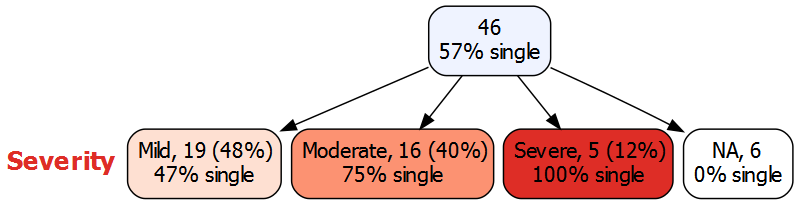Continuous variables such as `Score` can be dichotomized using notation such as `Score>10` or `Score<20`.

### Showing/hiding summaries on a node-by-node basis depending on the data in the node using the `runsummary` parameter

Sometimes it is desirable to show summaries only in nodes with certain characteristics. Consider the following example: suppose that in patients with a viral infection, the presence or absence of two features of the virus (Feature 1 and Feature 2) is recorded. To simulate this situation, let’s make up variables `Feature1` and `Feature2` but set them to NA if the `Viral` variable is FALSE or NA.

``````set.seed(1234)
FakeData\$Feature1 <- rbinom(nrow(FakeData),1,0.5)
FakeData\$Feature1[!FakeData\$Viral | is.na(FakeData\$Viral)] <- NA
FakeData\$Feature2 <- rbinom(nrow(FakeData),1,0.5)
FakeData\$Feature2[!FakeData\$Viral | is.na(FakeData\$Viral)] <- NA``````

Here is a tree for `Sex` and `Category` showing three summaries in each leaf node: the number and percent of patients with viral infections and number and percent of viruses with Feature 1 and with Feature 2.

``````vtree(FakeData,"Sex Category",sameline=TRUE,splitwidth=150,varminwidth=c(Category=6),
summary=c(
"Viral \nviral: %npct%%leafonly%",
"Feature1 , F1: %npct%%leafonly%",
"Feature2 , F2: %npct%%leafonly%"))``````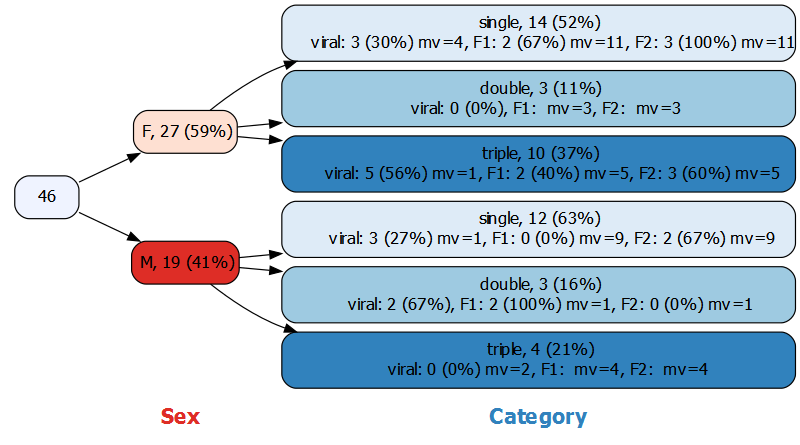But note that in two of the nodes there are no viral infections, so in these nodes there are no virus features to describe. The tree would be improved if we could turn off the summaries in nodes where there are no viral infections. We can do this by specifying, for each of the three summaries, a function that returns TRUE or FALSE for each node. The first function always returns TRUE: